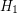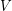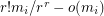# Osthus, Deryk

## Simultaneous partition of hypergraphs ★★

Author(s): Kühn; Osthus

Problem   Letandbe two-uniform hypergraph on the same vertex set. Does there always exist a partition ofintoclassessuch that for both, at leasthyperedges ofmeet each of the classes?

Keywords:

## Complexity of the H-factor problem. ★★

Author(s): Kühn; Osthus

An-factor in a graphis a set of vertex-disjoint copies ofcovering all vertices of.

Problem  Letbe a fixed positive real number anda fixed graph. Is it NP-hard to determine whether a graphonvertices and minimum degreecontains and-factor?

Keywords: